Next:9.7.3 Algorithm 3Up:9.7 Order StatisticsPrevious:9.7.1 Algorithm 1

## 9.7.2 Algorithm 2

Variation of quicksort.

Select (i, j, k) /* finds the kth element among A[i ...  A[j*/
{

pick a pivot element v;

partition A[i ...  A[j] so as to get

A[i ...  A[m - 1] with keys < v and

A[m ...  A[j] with keys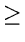v;

if(k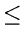m - 1)

Select (i, m - 1, k)

else

Select (m, j, k - m + i)

}

• Worst case complexity of the above algorithm is O(n2) (as in quicksort).
• Average Case:
• Note that select calls itself only once at a time whereas quicksort called itself twice each time.
• On an average, select calls itself on a subarray half as long as the subarray. To be conservative, suppose that each call of select is on an array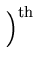the size on previous call. Then,
• T(nCn + T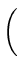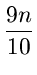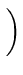which can be shown to be O(n).

eEL,CSA_Dept,IISc,Bangalore